Home | | Physics 12th Std | Example Solved Numerical Problems

# Example Solved Numerical Problems

Physics : Atomic and Nuclear Physics: Book Back, Exercise, Example Numerical Question with Answers, Solution: Example Solved Numerical Problems with Answers, Solution

Atomic and Nuclear Physics - Numerical Problems Questions with Answers, Solution

### Bohr atom model - Numerical Problems Questions with Answers, Solution

EXAMPLE 8.1

The radius of the 5th orbit of hydrogen atom is 13.25 Å. Calculate the wavelength of the electron in the 5th orbit.

Solution:

r = nλ

2 × 3.14 × 13.25Å = 5 × λ

λ = 16.64Å

EXAMPLE 8.2

Find the (i) angular momentum (ii) velocity of the electron in the 5th orbit of hydrogen atom. (h = 6.6 × 10–34 Js, m = 9.1 × 10–31 kg)

SolutionEXAMPLE 8.3

(a) Show that the ratio of velocity of an electron in the first Bohr orbit to the speed of light is a dimensionless number.

(b) Compute the velocity of electrons in ground state, first excited state and second excited state in Bohr atom model.

Solution

(a) The velocity of an electron in nth orbit isSubstituting for a0 in υn,where is the speed of light in free space or vacuum and its value is = 3 × 108 m s–1and α is called fine structure constant.

For a hydrogen atom, = 1 and for the first orbit, = 1, the ratio of velocity of electron in first orbit to the speed of light in vacuum or free space iswhich is a dimensionless number

α = 1/137

(b) Using fine structure constant, the velocity of electron can be written as

υn = αcZ / n

For hydrogen atom (= 1) the velocity of electron in nth orbit is

υn c/137 × 1/n = (2.19×106× 1/n  ms−1

For the first orbit (ground state), the velocity of electron is

υ = 2.19×106 ms−1

For the second orbit (first excited state), the velocity of electron is

υ2 = 1.095×106 ms−1

For the third orbit (second excited state), the velocity of electron is

υ3 = 0.73×106 ms−1

Here, υ1 > υ2 > υ3

EXAMPLE 8.4

The Bohr atom model is derived with the assumption that the nucleus of the atom is stationary and only electrons revolve around the nucleus. Suppose the nucleus is also in motion, then calculate the energy of this new system.

Solution

Let the mass of the electron be and mass of the nucleus be M. Since there is no external force acting on the system, the centre of mass of hydrogen atom remains at rest. Hence, both nucleus and electron move about the centre of mass as shown in figure.Let be the velocity of the nuclear motion and υ be the velocity of electron motion. Since the total linear momentum of the system is zero,

mυ + Mυ = 0 or

MV mυ = pSince the potential energy of the system is same, the total energy of the hydrogen can be expressed by replacing mass by reduced mass, which is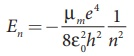Since the nucleus is very heavy compared to the electron, the reduced mass is closer to the mass of the electron.

### EXAMPLE 8.5

Suppose the energy of a hydrogen–like atom is given as En = − 54.4/n2 eV where nN . Calculate the following:

(a) Sketch the energy levels for this atom and compute its atomic number.

(b) If the atom is in ground state, compute its first excitation potential and also its ionization potential.

(c) When a photon with energy 42 eV and another photon with energy 56 eV are made to collide with this atom, does this atom absorb these photons?

(d) Determine the radius of its first Bohr orbit.

(e) Calculate the kinetic and potential energies in the ground state.

### Solutions

(a) Given that

En =− 54.4/n2 eV

For = 1, the ground state energy E1= –54.4 eV and for = 2, E2 = –13.6 eV.

Similarly, E3 = –6.04 eV, E4 = –3.4 eV and so on.

For large value of principal quantum number – that is, n = ∞, we get E = 0 eV.(b) For a hydrogen-like atom, ground state energy is

E1 = − 13.6/n2 Z2 eV

where is the atomic number. Hence, comparing this energy with given energy, we get, – 13.6 Z2 = – 54.4  = ±2. Since, atomic number cannot be negative number, = 2.

(c) The first excitation energy is

EI = E2 − E1 = −13.6eV −(−54.4 eV)

= 40.8eV

Hence, the first excitation potential is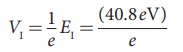= 40.8 volt

The first ionization energy is

Eionization = E − E1 = 0 −(−54.4 eV)

= 54.4 eV

Hence, the first ionization potential is

Vionization = 1/e Eionization = (54.4eV)/e

= 54.4 Volt

(d) Consider two photons to be A and B.

Given that photon A with energy 42 eV and photon B with energy 51 eV

From Bohr assumption, difference in energy levels is equal to photon energy, then atom will absorb energy, otherwise, not.

E2 − E1 = −13.6eV −(−54.4 eV)

= 40.8eV ≈ 41eV

Similarly,

E3 − E1 = −6.04 eV −(−54.4 eV)

= 48.36eV

E4 − E1 = −3.4 eV −(−54.4 eV)

= 51eV

E3 − E2 = −6.04 eV −(−13.6eV)

= 7.56eV

and so on.

But note that E2 – E1 ≠ 42 eV, E3 – E1 ≠ 42 eV, E4 – E1 ≠ 42 eV and E3 – E2 ≠ 42 eV.

For all possibilities, no difference in energy is an integer multiple of photon energy. Hence, photon A is not absorbed by this atom. But for Photon B, E4 – E1 = 51 eV, which means, Photon B can be absorbed by this atom.

(e) Since total energy is equal to negative of kinetic energy in Bohr atom model, we get

KEn = −En = −( − 54.4/n2 eV)

= 54.4/n2 eV

Potential energy is negative of twice the kinetic energy, which means,

Un = −2KEn = −2(54.4/n2 eV)

= −108.8/n2 eV

For a ground state, put n =1

Kinetic energy is KE1 = 54.4 eV and Potential energy is U1 = –108.8 eV

### Atomic and nuclear masses - Numerical Problems Questions with Answers, Solution

EXAMPLE 8.6

Calculate the average atomic mass of chlorine if no distinction is made between its different isotopes?

Solution

The element chlorine is a mixture of 75.77% of 3517Cl and 24.23% of 3717Cl . So the average atomic mass will be

(75.77/100) × 34.96885+ (24.23/100) × 36.96593u

= 35.453u

In fact, the chemist uses the average atomic mass or simply called chemical atomic weight (35.453 u for chlorine) of an element. So it must be remembered that the atomic mass which is mentioned in the periodic table is basically averaged atomic mass.

### Size and density of the nucleus - Numerical Problems Questions with Answers, Solution

EXAMPLE 8.7

Calculate the radius of 19779Au nucleus.

Solution

According to the equation (8.19),

= 1.2 × 10-15 × (197)1/3 = 6.97 × 10-15m

Or R = 6.97 F

### EXAMPLE 8.8

Calculate the density of the nucleus with mass number A.

Solution

From equation (8.19), the radius of the nuclei satisfy the equation = R0A1/3. Then the volume of the nucleus

= 4/3 πR3 = 4/3 πR3A

By ignoring the mass difference between the proton and neutron, the total mass of the nucleus having mass number is equal to A.m where is mass of the proton and is equal to 1.6726 x 10-27 kg.

### EXAMPLE 8.9

Compute the binding energy of 42He nucleus using the following data: Atomic mass of Helium atom, MA (He) = 4.00260 and that of hydrogen atom, mH = 1.00785u.

### Solution:

Binding energy BE = [ZmH Nmn A ]c2

For helium nucleus, = 2, A–Z = 4–2 = 2

Mass defect

= [(2×1.00785u) + (2 ×1.008665u) - 4.00260 u] ∆= 0.03038u

B.= 0.03038× c2

B.= 0.03038 × 931MeV = 28 MeV

[1uc2 = 931MeV ]

The binding energy of the  42He nucleus is 28 MeV.

### EXAMPLE 8.10

Compute the binding energy per nucleon of 42He nucleus.

### Solution

From example 8.9, we found that theof 42He =28 Mev

Binding energy per nucleon == 28 MeV/4 = 7 MeV.

EXAMPLE 8.11

(a) Calculate the disintegration energy when stationary 23292U nucleus decays to thorium 22890Th with the emission of α particle. The atomic masses are of 23292U = 232.037156 u , 22890Th = 228.028741u and 42He = 4.002603u

(b) Calculate kinetic energies of 22890Th and α-particle and their ratio.

Solution

The difference in masses

= (mU  mTh - mα)

= (232.037156–228.028741 – 4.002603)u

The mass lost in this decay = 0.005812 u

Since 1u = 931MeV, the energy Q released is

= (0.005812u)×(931MeV u)

= 5.41MeV

This disintegration energy Q appears as the kinetic energy of α particle and the daughter nucleus.

In any decay, the total linear momentum must be conserved.

Total linear momentum of the parent nucleus = total linear momentum of the daughter nucleus +α particle

Since before decay, the uranium nucleus is at rest, its momentum is zero.

By applying conservation of momentum, we getIt implies that the alpha particle and daughter nucleus move in opposite directions.

In magnitude mα υα = mThυTh

The velocity α particle υα =  [mTh/mα] υTh.

Note that mTh /ma > 1, so υα > υTh . The ratio of the kinetic energy of α particle to the daughter nucleusBy substituting, the value of υα into the above equation, we getThe kinetic energy of α particle is 57 times greater than the kinetic energy of the daughter nucleus (22890Th ).

The disintegration energy Q = total kinetic energy of products

K.Eα + K.ETh = 5.41MeV

57K.ETh K.ETh = 5.41MeV

K.ETh = 5.41/58 MeV = 0.093MeV

K.Eα = 57K.ETh = 57×0.093 = 5.301MeV

In fact, 98% of total kinetic energy is taken by the α particle.

### EXAMPLE 8.12

Calculate the number of nuclei of carbon-14 undecayed after 22,920 years if the initial number of carbon-14 atoms is 10,000. The half-life of carbon-14 is 5730 years.

### Solution

To get the time interval in terms of half- life,The number of nuclei remaining undecayed after 22,920 years,

= ( ½ )0 N0 = (1/2)4 ×10, 000

= 625

### EXAMPLE 8.13

A radioactive sample has 2.6 μof pure 137which has a half-life of 10 minutes (a) How many nuclei are present initially? (b) What is the activity initially? (c) What is the activity after 2 hours? (d) Calculate mean life of this sample.

### Solution

(a) To find N0, we have to find the number of 137N atoms in 2.6μg . The atomic mass of nitrogen is 13. Therefore, 13 g of 137N contains Avogadro number ( 6.02 ×1023 ) of atoms.

In 1 g, the number of 137N is equal to be 6.02×1023 / 13 atoms. So the number of 137N atoms in 2.6μg is

N = (6.02×1023/13) × 2.6×10−6 = 12.04×1016 atoms

(b) To find the initial activity R0, we have to evaluate decay constant λ

λ = 0.6931 / T1/2 = 0.6931 / 10×60 = 1.155×10−3 s−1

Therefore

R0 = λN0 = 1.155×10-3 ×12.04×1016

=13.90×1013 decays/s

= 13.90×1013 Bq

In terms of a curie,

R= 13.90×1015 / 3.7×1010 = 3.75×103 Ci

since 1Ci = 3.7×1010Bq

(c) Activity after 2 hours can be calculated in two different ways:

Method 1: R0e–λt

At = 2 hr = 7200 s

= 3.75 × 103 × e–7200 ×1.155 ×10–3

= 3.75 × 103 × 2.4 ×10–4 = 0.9 Ci

Method 2: = (1/2)n R0

Here = 120 min /10 min  = 12

= (1/2)12 ×3.75×103 ≈ 0.9Ci

(d) mean life τ = T1/2  / 0.6931 = 10×60 / 0.6931

= 865.67 s

### EXAMPLE 8.14

Keezhadi (கீழடி), a small hamlet, has become one of the very important archeological places of Tamilandu. It is located in Sivagangai district. A lot of tools, jewellery and charcoal, etc.) have been unearthed in Keezhadi which have given substantial evidence that an ancient urban civilization had thrived on the banks of river Vaigai. To determine the age of those materials, the charcoal of 200 g sent for carbon dating is given in the following figure (b). The activity of 146is found to be 38 decays/s. Calculate the age of charcoal.### Solution

To calculate the age, we need to know the initial activity (R0) of the characol (when the sample was alive).

The activity R of the sample

R0 e−λ      (1)

To find the time t, rewriting the above equation (1), eλt R0/R

By taking the logarithm on both sides, we get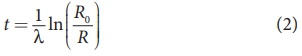Here = 38 decays/s=38 Bq.

To find decay constant, we use the equation[∴ 1yr = 365.25 × 24 × 60 × 60 s = 3.156 × 107 s]

λ = 3.83×10−12 s−1

To find the initial activity R0, we use the equation R0 = λN0 . Here N0 is the number of carbon-14 atoms present in the sample when it was alive. The mass of the characol is 200 g. In 12 g of carbon, there are 6.02 ×1023 carbon atoms. So 200 g contains,

[{ 6.02×1023 atoms / mol } / {12mol } ] × 200 ≈ 1×1025 Atoms

When the tree(sample) was alive, the ratio of 146to 126is 1.3 ×10-12 . So the total number of carbon-14 atoms is given by

N0 = 1×1025×1.3×10−12 = 1.3×1013 atoms

The initial activity

R0 = 3.83×10−12 ×1.3×1013 ≈ 50 decays / s

= 50 Bq

By substituting the value of R0 and λ in the equation (2), we getIn fact, the excavated materials were sent for carbon dating to USA by Archeological Department of Tamilnadu and the report confirmed that the age of Keezhadi artefacts lies between 2200 years to 2500 years (Sangam era- 400 BC to 200 BC). The Keezhadi excavations experimentally proved that urban civilization existed in Tamil Nadu even 2000 years ago!

### EXAMPLE 8.15

Calculate the amount of energy released when 1 kg of 93592undergoes fission reaction.

### Solution

235 g of  93592U  has 6.02 ×1023 atoms. In one gram of  93592, the number of atoms is equal to 6.02 ×1023 /235 = 2.56 ×1021 .

So the number of atoms in 1 kg of 93592= 2.56 ×1021 ×1000 = 2.56 ×1024.

Each 93592nucleus releases 200 MeV of energy during the fission. The total energy released by 1kg of 93592is

= 2.56 ×1024 × 200MeV = 5.12 ×1026 MeV

By converting in terms of joules,

= 5.12 ×1026 ×1.6 ×10-13 = 8.192 ×1013 .

In terms of Kilowatt hour,

Q = 8.192 ×1013 / 3.6 ×10= 2.27 ×107 kWh

This is enormously large energy which is enough to keep 100 W light bulb operating for 30,000 years. To produce this much energy through chemical reaction, around 20,000 tons of TNT(tri nitro toluene) has to be exploded.

Atomic and Nuclear Physics

Exercises

1. Consider two hydrogen atoms HA and HB in ground state. Assume that hydrogen atom HA is at rest and hydrogen atom HB is moving with a speed and make head-on collide on the stationary hydrogen atom HA. After the strike, both of them move together. What is minimum value of the kinetic energy of the moving hydrogen atom HB, such that any one of the hydrogen atoms reaches one of the excitation state.

[Ans: 20.4 eV]

Solution:2. In the Bohr atom model, the frequency of transitions is given by the following expression

Rc ( 1/n2 – 1/m2 ) , where n < m,

Consider the following transitions:Show that the frequency of these transitions obey sum rule (which is known as Ritz combination principle)

[Ans: v3→2  + v2→1 = v3→1]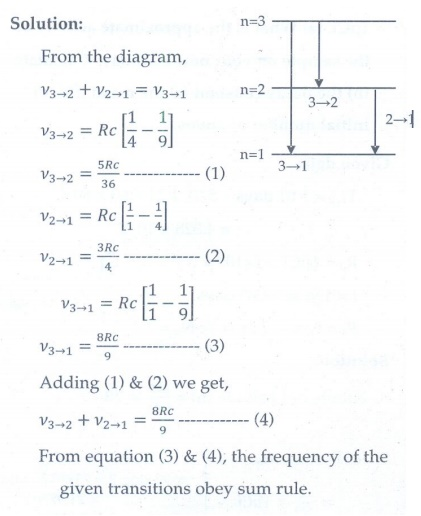3. (a) A hydrogen atom is excited by radiation of wavelength 97.5 nm. Find the principal quantum number of the excited state.

(b) Show that the total number of lines in emission spectrum is n(n-1) / 2 and  compute the total number of possible lines in emission spectrum.

[Ans: (a) n = 4 (b) 6 possible transitions]4. Calculate the radius of the earth if the density of the earth is equal to the density of the nucleus.[mass of earth 5.97 × 1024 kg ].

[Ans:180m]

Given data:

Mass of the earth = 5.97 x 1024 kg

Density of earth = Density of nucleus

= 2.3 x 1017 kg m-3

Solution: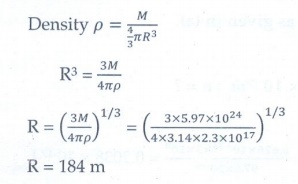5. Calculate the mass defect and the binding energy per nucleon of the 10847Ag nucleus. [atomic mass of Ag = 107.905949]

Ans: [∆= 0.990391and B.= 8.5MeV ]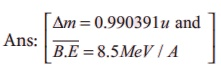Given data:

M = 107.905949 u

Z = 47      mH = 1.007825u

N = 61     m=1.008665 u

Solution:6. Half lives of two radioactive elements A and B are 20 minutes and 40 minutes respectively. Initially, the samples have equal number of nuclei. Calculate the ratio of decayed numbers of A and B nuclei after 80 minutes.

[Ans: 5:4]

Given data:

T1/2 of A = 20 min

T1/2 of B = 40 min ; Total time = 80 min

NOA = NOB

NOA - NA  / NOB - NB = ?

Solutions:

Decayed number of nuclei = No – NThe ratio of decayed numbers of A and B is 5 : 4.

7. On your birthday, you measure the activity of the sample 210Bi which has a half-life of 5.01 days. The initial activity that you measure is 1μCi . (a) What is the approximate activity of the sample on your next birthday? Calculate (b) the decay constant (c) the mean life (d) initial number of atoms.

[Ans: (a) 10-22 μCi (b) 1.6 ×10-6 s-1 (c) 7.24days (d) 2.31×1010 ]

Given data:

T1/2 = 5.01 days = 5.01 × 24 × 60 × 60 s

= 4.328 × 105 s

R= 1 μCi = 1 × 10-6 × 3.7 × 1010 Bq

t = 1 year = 365 days

Rt = ? ; λ=?; τ = ?  ; N= ?

Solution: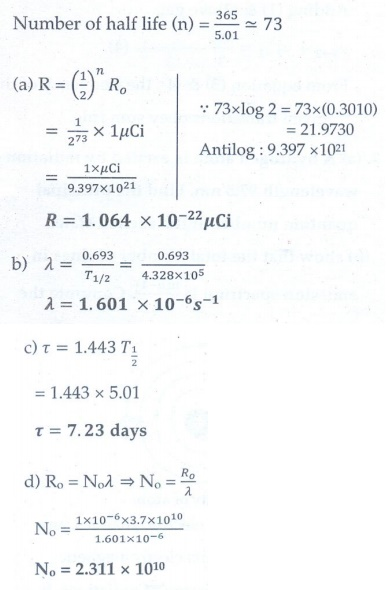8. Calculate the time required for 60% of a sample of radon undergo decay. Given T1/2 of radon =3.8 days

[Ans: 5.022 days]

Given data:

T1/2 = 3.8 days; λ= 0.693/3.8  per day

Amount of sample decayed = 60% of No

Amount of sample present (N) = 40% of No

No is initial amount of sample, t = ?

Solution:9. Assuming that energy released by the fission of a single 23592nucleus is 200MeV, calculate the number of fissions per second required to produce 1 watt power.

[Ans: 3.125 ×1010 ]

Given data:

Energy released during fission of single

23492U = 200MeV

Power = 1 watt = 1 J/s

No. of fissions per second required to produce

1W power = ?

Solution:

Energy produced = no. of fissions per second × energy released per fission

No.of fissions per second = energy produced / energy released per fission

= 1 / ( 200 ×106 ×1.6 ×10-19)

= 1/ (320 × 10-13)

= 1013/320 = 3.125 ×1010

No. of fissions per second = 3.125 × 1010

10. Show that the mass of radium ( 22688Ra ) with an activity of 1 curie is almost a gram. Given T1/2 =1600 years.

Given: T1/2 =1600 years (PTA-6)

Given data:

Ro = 1Ci= 3.7 × 1010  Bq

T1/2    = 1600 years

Mass of radium with an activity of 1 Ci= ?

Solution:The mass of radium with an activity of 1 Ci is almost a gram.

11. Characol pieces of tree is found from an archeological site. The carbon-14 content of this characol is only 17.5% that of equivalent sample of carbon from a living tree. What is the age of tree?

[Ans: 1.44 × 104 yr]

Given data:

The amount of 14C present (N) = 17.5% of No

T1/2 = 5730 years

Age of the tree (t) = ?

Solution: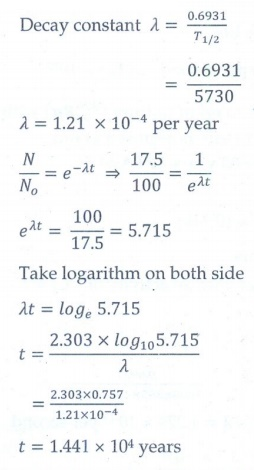Tags : with Answers, Solution | Atomic and Nuclear Physics | Physics , 12th Physics : UNIT 9 : Atomic and Nuclear Physics
Study Material, Lecturing Notes, Assignment, Reference, Wiki description explanation, brief detail
12th Physics : UNIT 9 : Atomic and Nuclear Physics : Example Solved Numerical Problems | with Answers, Solution | Atomic and Nuclear Physics | Physics